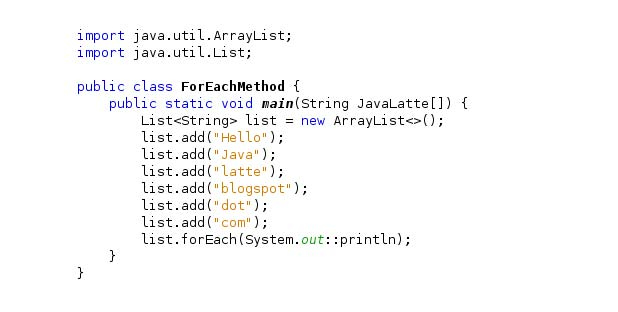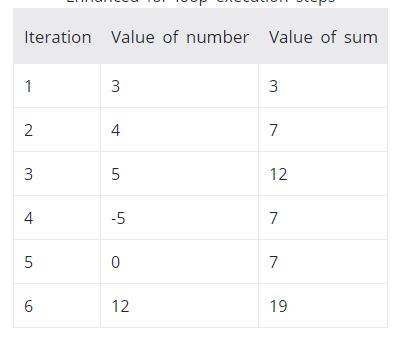# blog posts# In Java, there is another form of loop (in addition to the standard for loop) for working with arrays and sets.

If you are working with arrays and sets, you can use another for loop structure (advanced for loop form) to duplicate their items.

This type of loop is called for-each because the loop is repeated through each array / set element.

Here is an example of repeating elements of an array using the standard for loop in Java:

1. class ForLoop {
2. public static void main (String [] args) {
3. char [] vowels = {‘a’, ‘e’, ​​’i’, ‘o’, ‘u’};
4. for (int i = 0; i <vowels.length; ++ i) {
5. System.out.println (vowels [i]);
6. }
7. }
8. }

#### You can also write the above code using the for-each loop:

1. class AssignmentOperator {
2. public static void main (String [] args) {
3. char [] vowels = {‘a’, ‘e’, ​​’i’, ‘o’, ‘u’};
4. // foreach loop
5. for (char item: vowels) {
6. System.out.println (item);
7. }
8. }
9. }

#### The output of both codes is similar and equal to:

1. a
2. e
3. i
4. o
5. u

Using the advanced for loop is easier to write and makes the code more readable. Hence it is usually recommended more than the standard form.

## For-each ring structure

First let’s look at the for-each loop structure:

for (data_type item: collection) {

}

In the above structure,

• collection is a collection or array on which you want to write a circle.
• item is a single element of the collection.

## How does the for-each loop work?

Here’s how the for-each loop works.

• Repeat through any element in the given array or collection,
• Stores each item in an item.
• And executes the body of the ring.

Example: for-each loop

The following program calculates the sum of all the elements of an array of integers.

1. class EnhancedForLoop {
2. public static void main (String [] args) {
3. int [] numbers = {3, 4, 5, -5, 0, 12};
4. int sum = 0;
5. for (int number: numbers) {
6. sum + = number;
7. }
8. System.out.println (“Sum =” + sum);
9. }
10. }

Output

Sum = 19

In the above program, the execution of the for-each loop is as follows:You see a repeat of the for-each loop

• All elements of numbers are repeated.
• Each element is stored in the number variable.
• The body of the loop is executed, ie the number is added to the sum.